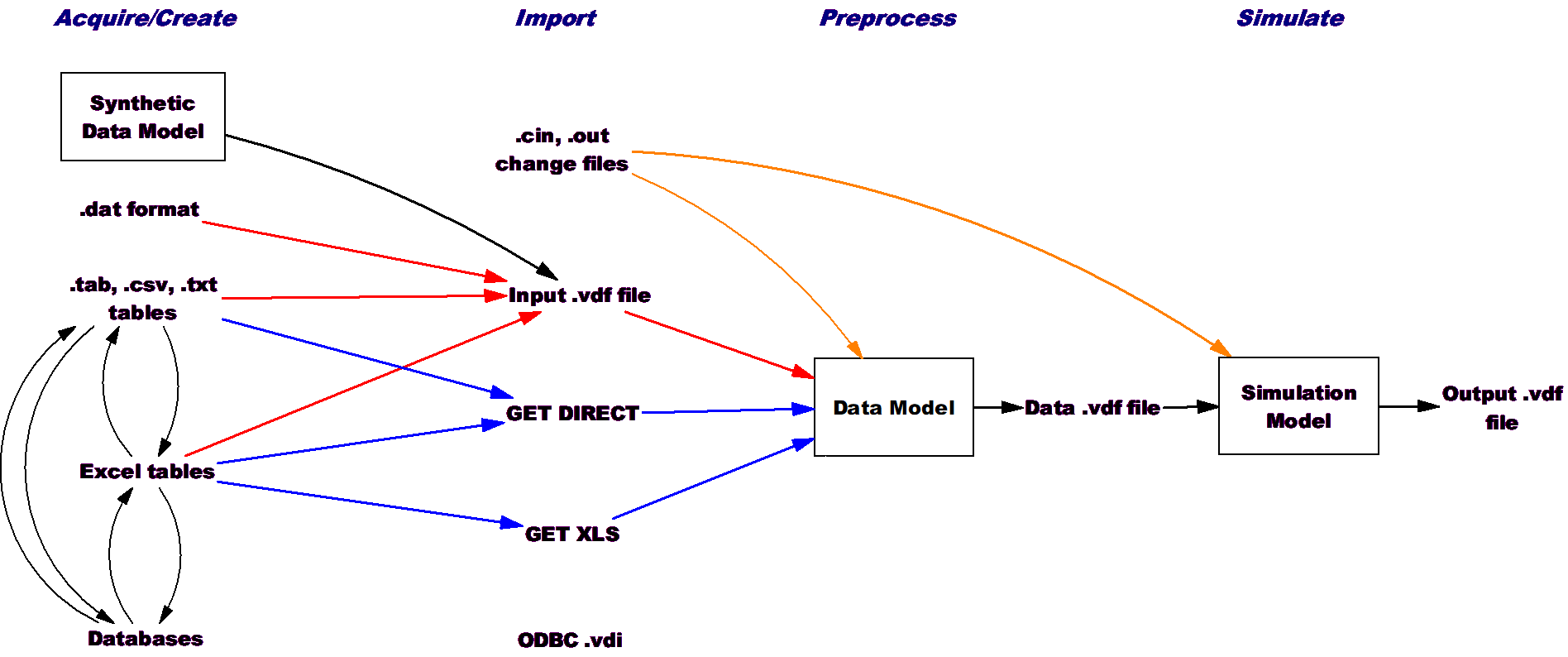# Vensim Help

 Navigation: Reference Guide > Functions > Dynamic Functions TREND(input, average time,initial trend) Compute the TREND of input

Returns the average fractional growth rate (negative for decline) in the input.  Equivalent to the equations:

TREND=ZIDZ(input-avval,average time*ABS(avval))

avval=INTEG((input-avval)/average time,input/(1+ini*averate time))

The TREND function provides a very simple trend estimate for a variable.  It really only makes sense for numbers that are always positive and don’t get very close to zero.  If you want to trend a number that may be positive or negative the TREND function is not very useful.  Instead, you should use the fractional trend relative to a quantity that is always positive (for example change in profit as a fraction of gross income).

Units: TREND(units,time,1/time) --> 1/time

Units for the average time must match those of TIME  STEP.

Example

R = 10 + RAMP(1,30,70)

TR = TREND(R,5,.08)